You are at the newest post.

### 21 GIFs That Explain Mathematical Concepts

var imagebase='file://D:/Program Files (x86)/FeedReader/'; 21 GIFs That Explain Mathematical Concepts 15:55 27.08.2014, Maxx, amazing, World Of Technology “Let's face it; by and large math is not easy, but that's what makes it so rewarding when you conquer a problem, and reach new heights of understanding.”

Danica McKellar

As we usher in the start of a new school year, it’s time to hit the ground running in your classes! Math can be pretty tough, but since it is the language in which scientists interpret the Universe, there’s really no getting around learning it. Check out these gifs that will help you visualize some tricky aspects of math, so you can dominate your exams this year.

Ellipse:Solving Pascal triangles:Use FOIL to easily multiply binomials:Here’s how you solve logarithms: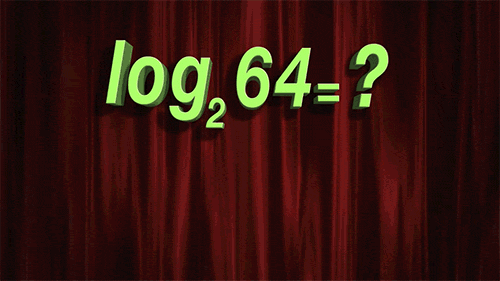Use this trick so you don’t get mixed up when doing matrix transpositions:What the Pythagorean Theorem is really trying to show you:Exterior angles of polygons will ALWAYS add up to 360 degrees:If you’re studying trig, you better get pretty comfortable with circles. Check out this visualization that shows what you’re really looking at when you deal with pi: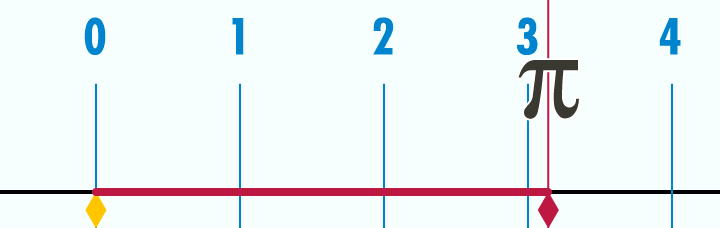If an arc of a circle is the same length as its radius, the resulting angle is one radian:Visualizing sine (red) on the Y axis and cosine (blue) on the X axis. The relative position of the circle is shown in black: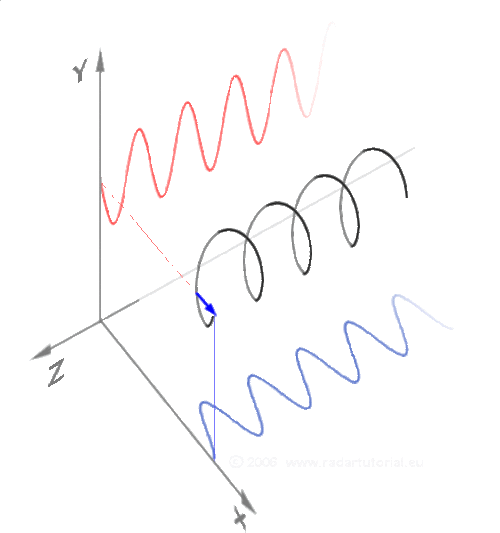This shows the same thing, but a bit more simply:Here’s how sine and cosine apply to triangles:Cosine is the derivative of sine: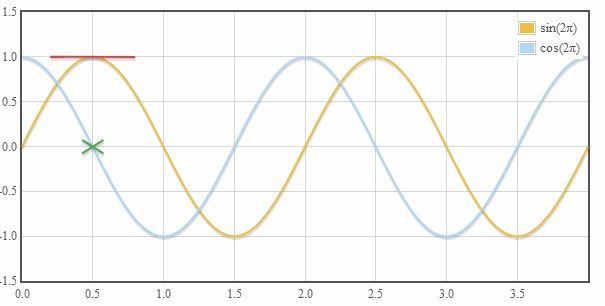Tangent lines:Flipped on its side, the shape begins to make more sense:Converting a function from Cartesian to Polar coordinates: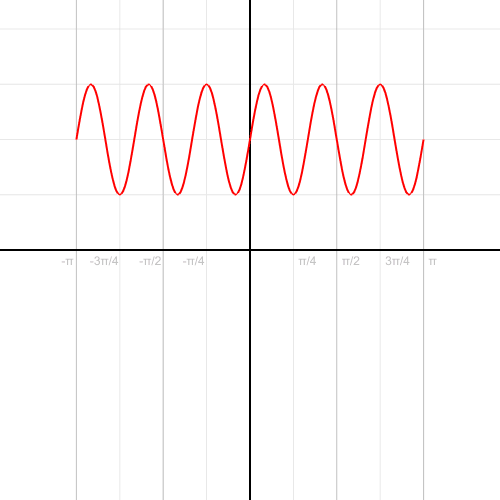Drawing a parabola:The Riemann sum is the approximate area under a curve:Hyperbola:Translating that into 3D, you get a hyperboloid. Believe it or not, it’s made with completely straight lines:Seriously. You can even make it do this: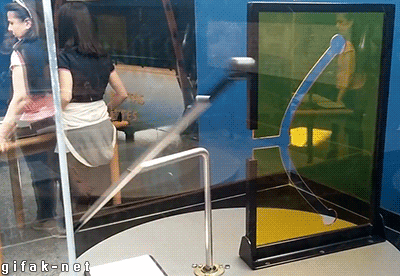## Don't be the product, buy the product!YES, I want to SOUP ●UP for ...